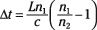##### Optics For Dummies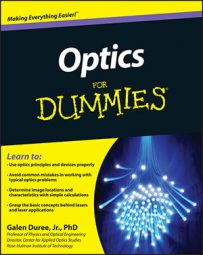Optics covers the study of light. Three phenomena — reflection, refraction, and diffraction — help you predict where a ray or rays of light will go. Study up on other important optics topics, too, including interference, polarization, and fiber optics.

## Reflection and refraction equations for predicting light's direction

Reflection and refraction are two processes that change the direction light travels. Using the equations for calculating reflection and refraction, you can predict where rays encountering a surface will go — whether they reflect or refract (bounce off the surface or bend through it) — which is an important concept in the study of optics. The following equations help you determine reflection and refraction angles:

• The law of reflection: The law of reflection shows the relationship between the incident angle and the reflected angle for a ray of light incident on a surface. The angles are measured relative to the surface normal (a line that is perpendicular to the surface), not relative to the surface itself. Here’s the formula: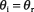• The index of refraction: This quantity describes the effect of atoms and molecules on the light as it travels through a transparent material. Use this basic formula for the index of refraction: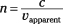• Snell’s law or the law of refraction: Snells law shows the relationship between the incident angle and the transmitted angle (refracted angle) for a ray of light incident on a surface of a transparent material. You can see how Snell’s law works in the following formula: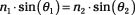• The critical angle for total internal reflection: Total internal reflection is the situation where light hits and reflects off the surface of a transparent material without transmitting through the surface. It utilizes the critical angle (the minimum angle of incidence where total internal reflection takes place.). For total internal reflection to occur, the light must start in the material with the higher index. Here’s the formula: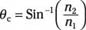## Equations for optical imaging

Imaging is a key function of optics. Specific optics equations can help you determine the basic characteristics of an image and predict where it will form. Use the following optics equations for your imaging needs:

• Lateral magnification: Lateral magnification is one way you can describe how big the image is compared to the original object. Here are the equations: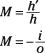• Locating images formed by mirrors: An object placed a certain distance away from a mirror will produce an image at a certain distance from the mirror. In some cases where the mirrors are curved, you may be given the focal length of a mirror. Use these equations: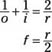• Location of images formed by a refracting surface: An object placed a certain distance away from a refracting surface will produce an image at a certain distance from the surface. The equation for this is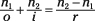• The lens maker’s formula: This equation allows you to calculate the focal length of a lens if all you know is the curvature of the two surfaces. Here’s the lens maker’s formula: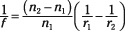• The thin lens equation: An object placed a certain distance away from a lens will produce an image at a certain distance from the lens, and the thin lens equation relates the image location to the object distance and focal length. The following is the thin lens equation: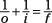## Optical polarization equations

Optical polarization is the orientation of the planes of oscillation of the electric field vectors for many light waves. Optical polarization is often a major consideration in the construction of many optical systems, so equations for working with polarization come in handy. The following equations highlight some important polarization concepts. The equations listed here allow you to calculate how to make polarized light by reflection and to determine how much light passes through multiple polarizers:

• Polarizing angle or Brewster’s angle: This angle is the angle of incidence where the reflected light is linearly polarized. Here’s the equation: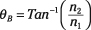• Malus’ law: This equation allows you to calculate how much polarized light passes through a linear polarizer. The equation for Malus’ law is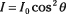• Phase retardation in a birefringent material: A birefringent material has two indexes of refraction. When you send polarized light into a birefringent material, the two components travel through the material with different speeds. This discrepancy can result in a change in the polarization state or simply rotate the polarization state. Use this equation: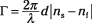## Optical interference equations

Optical interference is just the interaction of two or more light waves. Optical interference is useful in many applications, so you need to understand some basic equations related to this optical phenomenon. The following equations allow you to calculate various quantities related to optical interference in the two most common interference arrangements.

• The location of the bright and dark fringes in Young’s two-slit interference arrangement: The following equations allow you to calculate the location of the bright fringes (where constructive interference occurs) and dark fringes (where destructive interference occurs):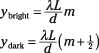• The phase shift due to the film thickness in thin film interference: When light is incident straight onto a thin film (such as an oil slick on the surface of a pool of water), light rays reflecting from the top and the bottom of the film interfere (either constructively or destructively depending on the film thickness and the wavelength of the light). The following equations determine constructive or destructive interference depending on whether the phase shift produced by reflection needs to be shifted by half the wavelength (the first equation) or maintained (the second equation):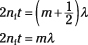## Optical diffraction equations

Diffraction is light’s response to having something mess with its path, so diffraction occurs only when something blocks part of the wavefront. Diffraction is the phenomenon where light bends around an obstacle (this bending is not due to refraction, because the material doesn’t change as refraction requires). The following equations cover the most common situations involving diffraction, including resolution.

• Resolution: Resolution is the minimum angular separation between two objects such that you can tell that there are two distinct objects. Here’s the equation for determining resolution: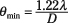The location of the dark fringes produced by diffraction through a single slit: Because a slit has a width larger than the wavelength, light rays from different parts of the slit interfere with each other, creating a fringe pattern. You can relatively easily locate the points where the light destructively interferes by using the following equation: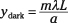• The location of the different diffraction orders from a diffraction grating: A diffraction grating has a very large number of slits spaced closely together, such that the light from each of these slits interferes with the light from the others. You can pretty easily identify where the light constructively interferes by using the following equation: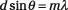## Equations for the characteristics of fiber-optics fibers

Besides imaging, fiber-optic networks are probably the largest application of optics. Fiber optics are very long, thin glass fibers that transfer information-bearing light from one place to another, but that may not be in direct sight of each other. You need to be aware of a few characteristics of the particular fiber you’re using so that you can ensure the information is accurately transmitted from one end of the fiber to the other. The following equations cover three of the basic parameters necessary for proper use of optical fibers.

• The maximum acceptance angle for a fiber: This angle is the largest angle of incidence at which light can enter the end of the fiber and be totally internally reflected inside the fiber. Angles of incidence larger than this angle will transmit through the sides of the fiber and not make it to the other end. The equation for this angle is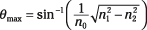• The numerical aperture for a fiber: The numerical aperture is a measure of the light-gathering power of the fiber. It has a maximum value of 1 (all the light remains trapped inside the fiber) and a minimum value of 0 (only light incident at an angle of 0 degrees on the end of the fiber remains trapped in the fiber). Use this equation: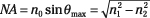• Intermodal dispersion in a fiber: This characteristic measures the difference in time that different fiber modes take to reach the end of the fiber. The larger this time difference, the shorter the fiber has to be so that the information on this light doesn’t turn into junk. Here’s the equation: